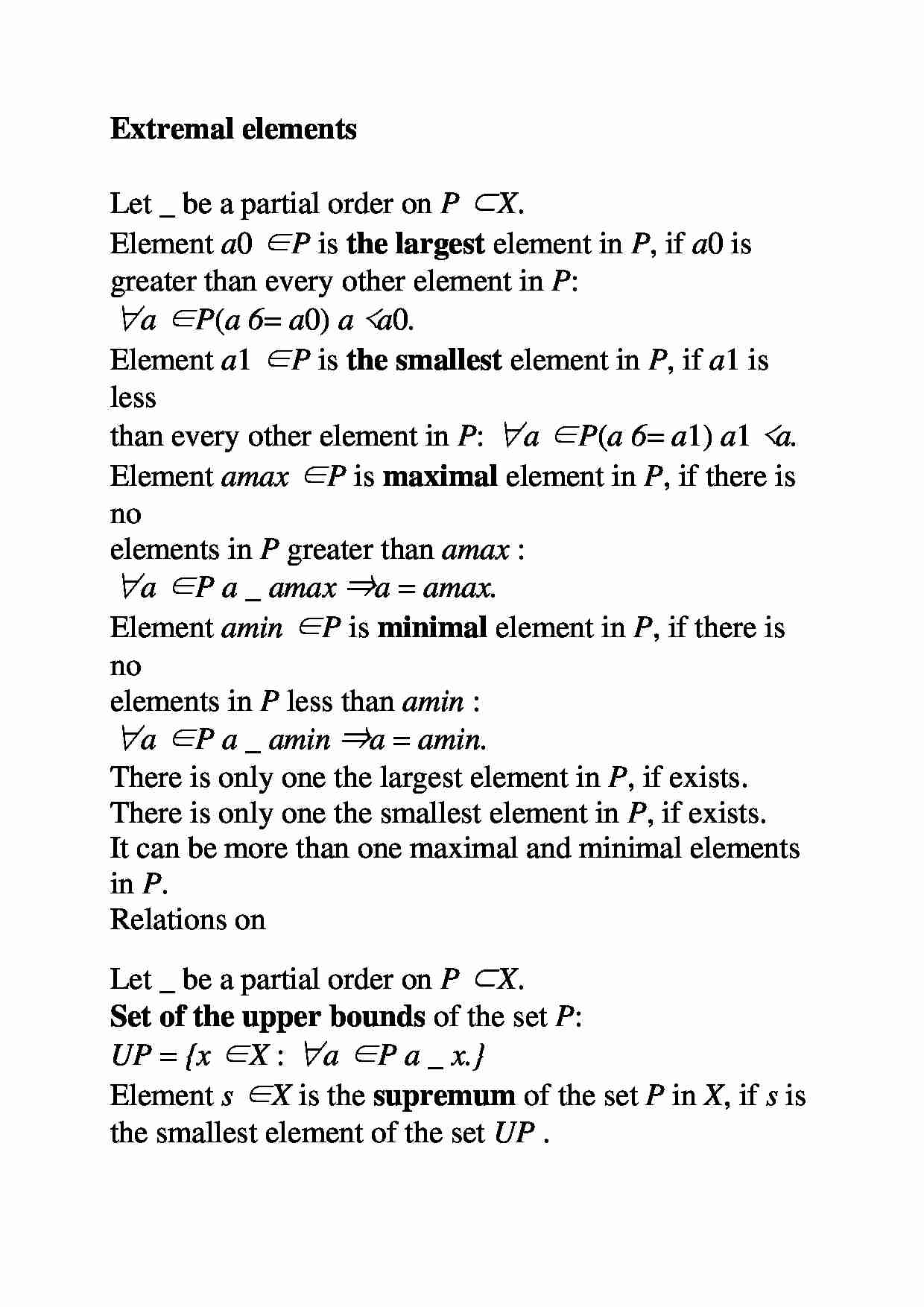# Elementy ekstremalne

Nasza ocena:

Wyświetleń: 770
Komentarze: 0

### Pobierz ten dokument za darmo

Podgląd dokumentu## Fragment notatki:

Extremal elements Let _ be a partial order on P ⊂ X . Element a 0 ∈ P is the largest element in P , if a 0 is greater than every other element in P : ∀ a ∈ P ( a 6 = a 0 ) a ≺ a 0 . Element a 1 ∈ P is the smallest element in P , if a 1 is less than every other element in P : ∀ a ∈ P ( a 6 = a 1 ) a 1 ≺ a. Element a max ∈ P is maximal element in P , if there is no elements in P greater than a max : ∀ a ∈ P a _ a max ⇒ a = a max . Element a min ∈ P is minimal element in P , if there is no elements in P less than a min : ∀ a ∈ P a _ a min ⇒ a = a min . There is only one the largest element in P , if exists. There is only one the smallest element in P , if exists. It can be more than one maximal and minimal elements in P . Relations on Let _ be a partial order on P ⊂ X . Set of the upper bounds of the set P : U P = { x ∈ X : ∀ a ∈ P a _ x. } Element s ∈ X is the supremum of the set P in X , if s is the smallest element of the set U P . s = supP ⇔ ∀ a ∈ P a _ s ∧ ( ∀ s ′ ∈ U P a _ s ′ ⇒ s _ s ′ ) . Set of the lower bounds of the set P : L P = { x ∈ X : ∀ a ∈ P x _ a. } Element q ∈ X is the infimum of the set P in X , if q is the largest element of the set L P . q = infP ⇔ ∀ a ∈ P q _ a ∧ ( ∀ q ′ ∈ L P q ′ _ a ⇒ q ′ _ q ) . Supremum and infimum of P don't have to belong to P . Relations on Example: Let X = P ( N ) - set of all subsets of N , ordered by the subset relation ⊂ . Let P = {{ n } : n ∈ N } - set of all 1-element subsets of N . - The largest element in P doesn't exist. (It would have to be the 1-element set containing all others 1-element sets.) - The smallest element in P doesn't exist. (It would have to be the 1-element set contained in every other 1-element set.) - Maximal elements - every { n } . (There is nothing ”greater” in the sense of subset relation.) - Minimal elements - every { n } . (There is nothing ”smaller” in the sense of subset relation.) Relations - Set of the upper bounds in X : U P = { N } . (Only N contains all the 1-element sets.) So: supP = N . - Set of the lower bounds in X : L P = { ? } . (Only ? is ... zobacz całą notatkę

## Komentarze użytkowników (0)

Zaloguj się, aby dodać komentarz